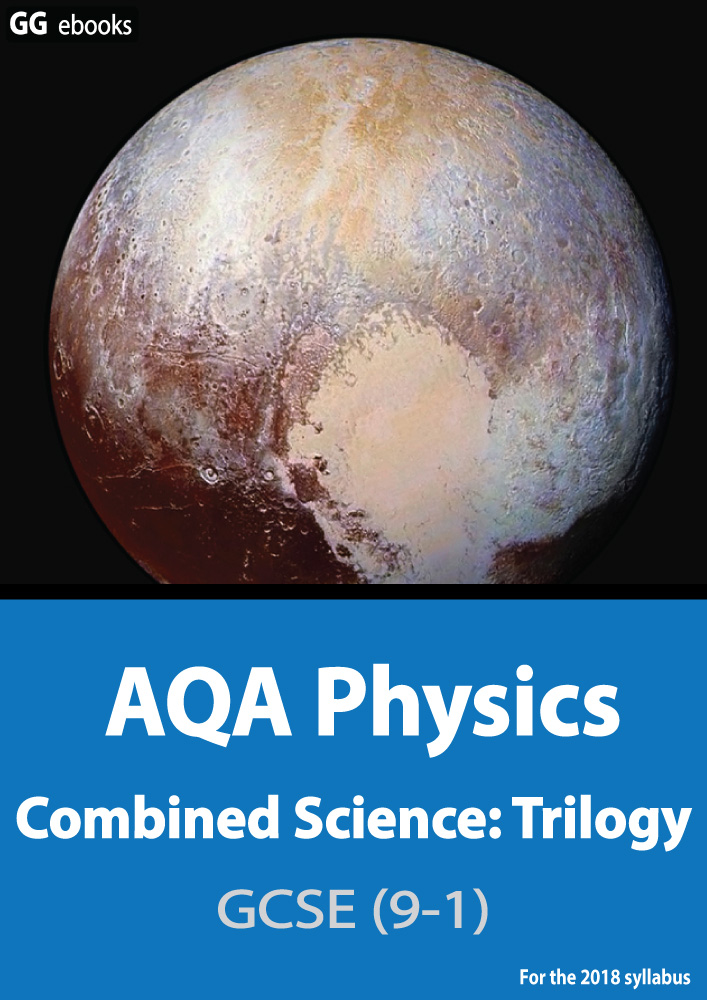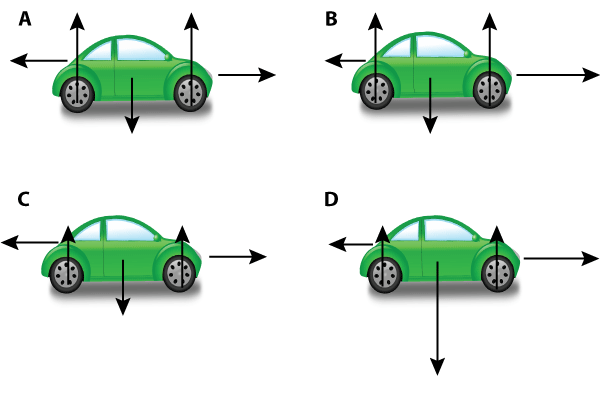NEED HELP? AQA GCSE digital textbooks From the makers of Grade Gorilla GGebooks.com(Opens in a new tab)Forces, Vectors, Weight, Work, Stretching. 1. Which of these sentences is correct? A). You are weightless on the moon B). Your mass changes as you travel into space C). Your weight is a measure of the density of your body D). Your weight is the pull of gravity on you. ? A B C D On Mars the gravitational field strength is 4 N/kg.2. What is the weight of a 60kg astronaut on Mars? A). 60N B). 240kg C). 15N D). 240N ? A B C D 3. What is the mass of a Mars rock of weight 2kN? A). 8 kg B). 8000N C). 50 N D). 500kg ? A B C D 4. When taking a mars rock into deep space, which of these quantities changes, if any? A). mass changes, weight stays the same B). weight changes, mass stays the same C). both mass and weight stay the same D). both mass and weight change ? A B C D Choose the correct units for the following measurements : 5. Friction. ? joules newtons kilograms m/s s N/m watts 6. Work done. ? joules newtons kilograms m/s s N/m watts 7. Which of the following are vector quantities? A). mass and velocity B). acceleration and force C). mass and displacement D). velocity and time ? A B C D 8. Which of the following are scalar quantities? A). mass and velocity B). acceleration and velocity C). mass and time D). velocity and time ? A B C D 9. A remote controlled car is switched on and accelerates from rest, moving left to right. Which of these 4 free body diagrams correctly shows the relative sizes of the forces acting on the car?? A B C D